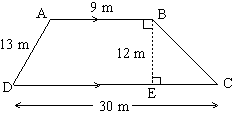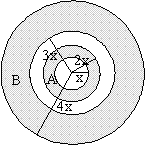# PERSONAL INTERVIEWCat Exam
Explore more-CAT
KI Unique
Test Yourself
Help

## KI UNIQUE QUANTITATIVE SECTION:

The Quantitative Ability Section tests the ability of problem solving in a candidate. This section covers various topics from Mathematics and Data sufficiency in general. Often this section creates a fearful impression on the students. Moreover the students have the pressure to score in it heavily. That is why its tips relevance is that much more for the students.

### BASIC TIPS

1. Numbers, Geometry and Algebra account for a big chunk of questions in CAT, and they deserve due respect.
2. The total quant area can be divided into 4 main parts:

### PARTS DIVISION:QUANT SECTION• Part 1: Numbers, and Geometry and Mensurations -- These two topics have to be considered a separate area, because the maximum number of questions come from here.
• Part 2:Equations, Ratio Proportion and Variation, Percentages Profit and Loss, Averages Mixtures and Alligations, Simple Interest and Compound Interest, Time and Work, and Time and Distance.
• Part 3:Indices, Logs and Surds, Quadratic Equations, Progressions, Special Equations and Inequalities.
• Part 4: Pure Maths -- Permutations and Combinations, Functions and Graphs, Number Systems, Coordinate Geometry.

### SPECIFIC TIPS:

• Learn your mathematical tables up to 30, square roots and cube roots. These assist in minimizing the calculation time required. Memorize all the rules of easy solving techniques you come across.
• Be very careful with the units used. There may be a need to do conversions to get an accurate answer, so learn these, especially if the multiple-choice answers are given in a different unit altogether.
• Improve your speed in calculation:80% of the time is spent on cracking the concept in any question, 20% is spent on calculation. To ensure speedier calculation, everyday in the morning (for at least five days a week) one must practice calculations like addition, subtraction, multiplication, and division for about 10 minutes. This practice can save a lot of precious time in the actual exam
• Even Quant section tests reasoning ability. So, don’t misinterpret it as another section.
Here is an example of CAT question testing reasoning ability:

### EXAMPLES:

Quantitative Comparisons

1. Quantity A : (-6)4
Quantity B : (-6)5
• if the
• if the
• if the two quantities are equal;
• if the
Ans: A

2. Quantity A: Time to travel 95 miles at 50 miles per hour
Quantity B: Time to travel 125 miles at 60 miles per hour
• Quantity A is greater
• Quantity A equals
• Quantity B is greater
• Relationship Indeterminate
Ans: C

### OTHER SAMPLE QUESTIONS

1. Tom, Dick and Harry went for lunch to a restaurant. Tom had \$100 with him, Dick had \$60 and Harry had \$409. They got a bill for \$104 and decided to give a tip of \$16. They further decided to share the total expenses in the ratio of the amounts of money each carried. The amount of money which Tom paid more than what Harry paid is

• 120
• 200
• 60
• 24
• 36
• Ans: E

2. A plot of land is in the shape of a trapezium whose dimensions are given in the figure below :• 50 m
• 64 m
• 72 m
• 84 m
• None of the above
• Ans : C

3. Four concentric ( having the same center ) circles with radii, x, 2x, 3x and 4x are drawn to form two rings A and B as shown in the figure.Ratio of the area of inner ring A to the area of outer ring B is

• 1 : 2
• 1 : 4
• 2 : 3
• 3 : 7
• None of the above
• Ans : D

4. If 3/p = 6 and 3/q = 15 then p - q = ?

• 1/3
• 2/5
• 3/10
• 5/6
• None of the above
• Ans : C

5. A father is three times as old as his son. After fifteen years the father will be twice as old as his son's age at that time. Hence the father's present age is

6. 36
7. 42
8. 45
9. 48
10. None of the above
11. Ans : C

### DATA SUFFICIENCY

DS can come under any section. But we have categorized it under Quantitative Section.

• The examinee basically has to decide whether or not he/she has enough information in hand to answer a given question.
• The examinee must be through with all the alternatives of data sufficiency so that he may decide quickly for the choice of the related alternative.
• Be careful not to carry over any information from one numbered statement to another. (Making this mistake is remarkably easy, especially under time pressure and in a momentary lapse of concentration.)
• If a question asks for a numerical value (as opposed to a quantitative expression that includes variables), the question is answerable only if a numbered statement (1 or 2) yields one and only one possible numerical answer--not a range of values.
• In distinct contrast to Problem Solving geometry figures, Data Sufficiency figures are not necessarily drawn proportionately--unless a figure indicates explicitly that it is drawn to scale. Do NOT rely on your eye to measure angle sizes, line segment length, or areas.

Example of Data sufficiency question-

Is the product of x and y greater than 60?

• The sum of x and y is greater than 60.
• Each of the variables is greater than 2.
Ans: Statement 1 by itself is not sufficient. One of the two variables could be a small fraction. (Think of 500 and 0.01.) Statement 2 by itself could yield the product of 3 and 4. However, the information from both statements is taken together; we can definitively state that the answer to this question is "true.
Copy Right © 2012 by Knowledge Icon
Website Designed & Developed By Dream Business Systems